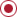Keywords Last Name

##Regression Analysis

"Regression Analysis" is a descriptor in the National Library of Medicine's controlled vocabulary thesaurus, MeSH (Medical Subject Headings). Descriptors are arranged in a hierarchical structure, which enables searching at various levels of specificity.

Procedures for finding the mathematical function which best describes the relationship between a dependent variable and one or more independent variables. In linear regression (see LINEAR MODELS) the relationship is constrained to be a straight line and LEAST-SQUARES ANALYSIS is used to determine the best fit. In logistic regression (see LOGISTIC MODELS) the dependent variable is qualitative rather than continuously variable and LIKELIHOOD FUNCTIONS are used to find the best relationship. In multiple regression, the dependent variable is considered to depend on more than a single independent variable.

This graph shows the total number of publications written about "Regression Analysis" by people in this website by year, and whether "Regression Analysis" was a major or minor topic of these publications.To see the data from this visualization as text, click here.
_
_